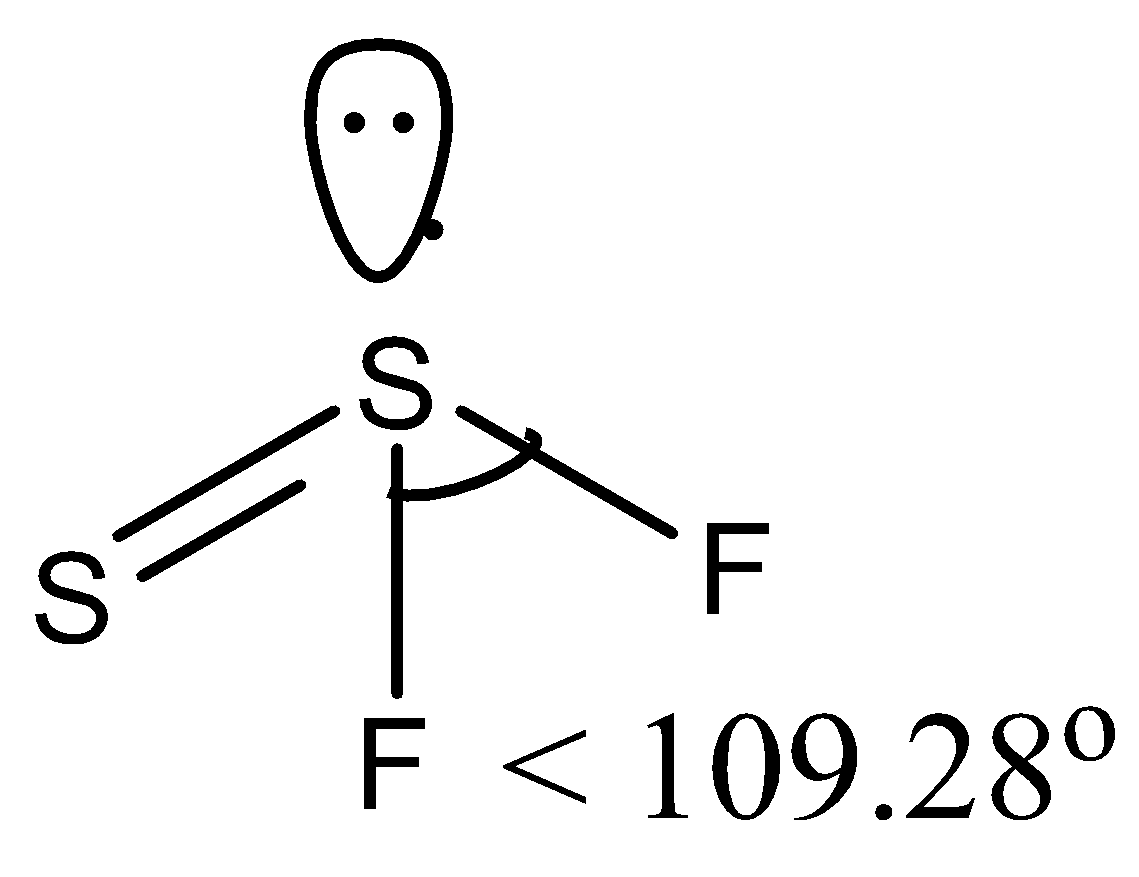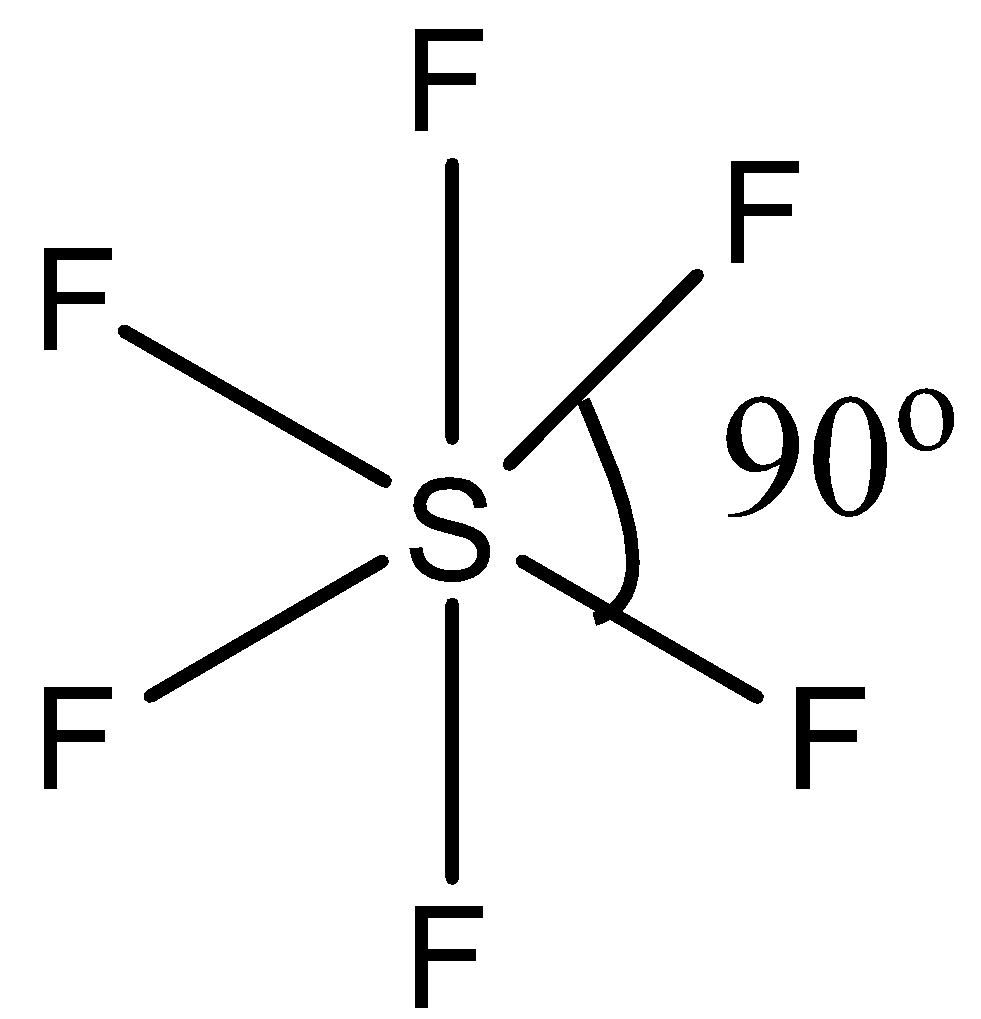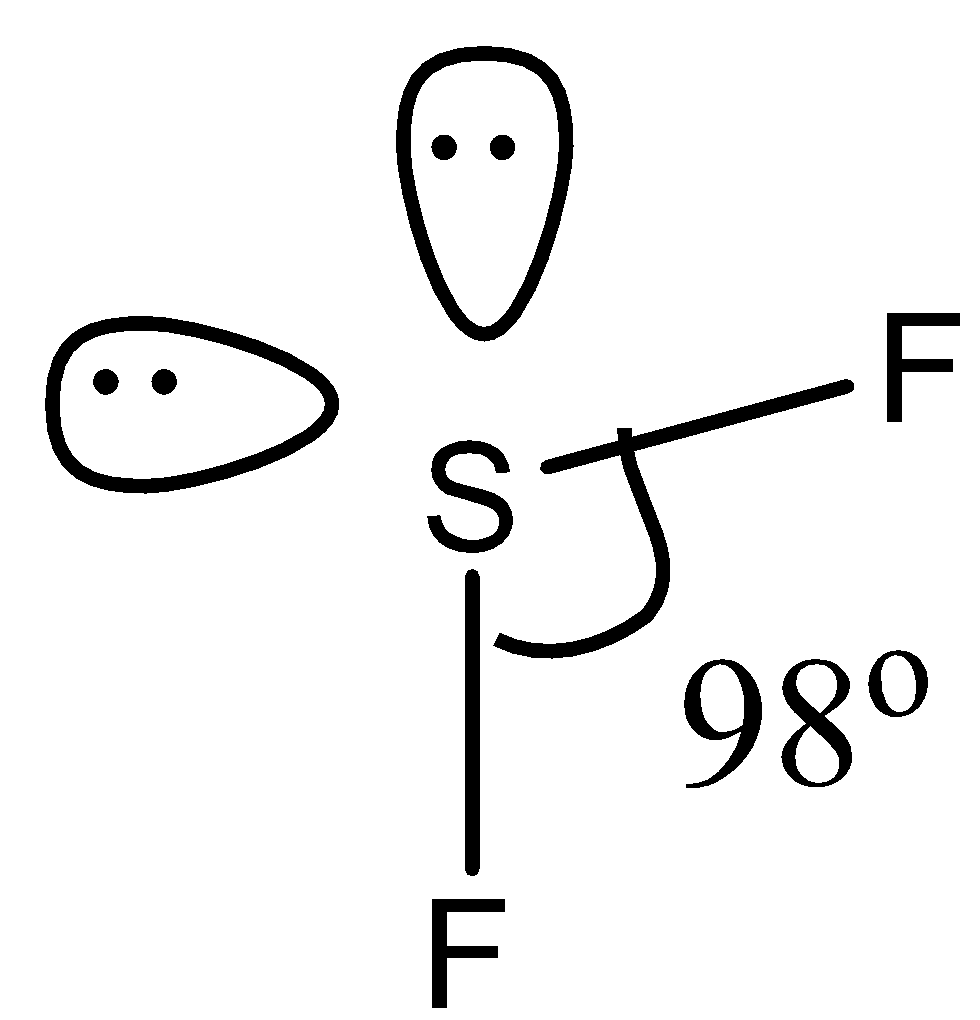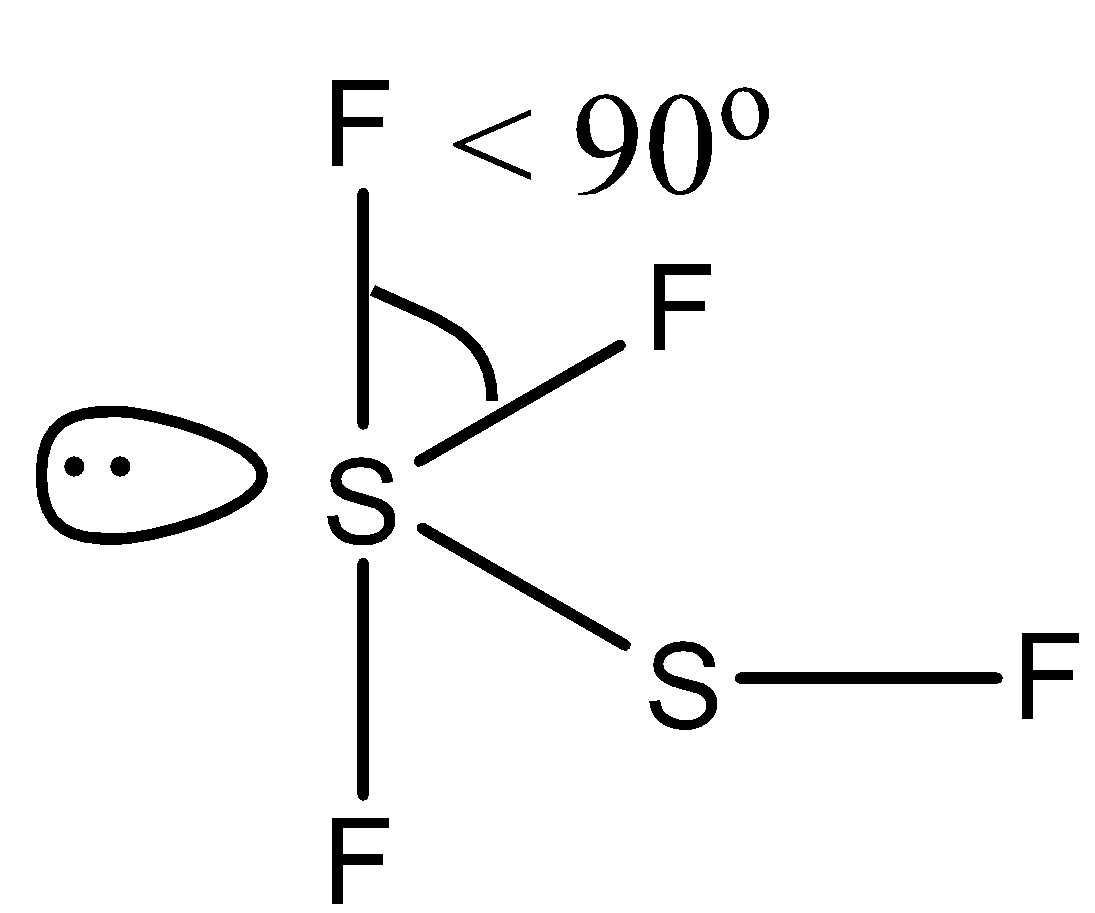Minimum $F-S-F$ bond angle is present in:(a)- $SS{{F}_{2}}$ (b)- $S{{F}_{6}}$ (c)- $S{{F}_{2}}$ (d)- ${{F}_{3}}SSF$Verified
119.4k+ views
Hint: Bond angle can be measured as the angle between three atoms, keeping one atom as a central atom and it is forming a bond with the other two atoms. The molecule that has many atoms will have a small bond angle because of steric hindrance.

The bond angle can be measured as the angle between three atoms, keeping one atom as the central atom and it is forming a bond with the other two atoms. So let us examine all the compounds in the options one by one.
(a)- $SS{{F}_{2}}$, this compound is also written as ${{S}_{2}}{{F}_{2}}$ and is known as disulfur difluoride. It has two isomers, so the structure of the isomer that has an $F-S-F$bond is given below:So there is a lone pair on the sulfur atom so the bond angle is reduced from the tetrahedral angle. So it has $<\text{ 10}{{\text{9}}^{\circ }}\text{28 }\!\!'\!\!\text{ }$ bond angle.
(b)- $S{{F}_{6}}$, this compound is sulfur hexafluoride. This compound has regular octahedral geometry. So its structure is given below:Since it has a symmetric structure, the bond angle is ${{90}^{\circ }}$.
(c)- $S{{F}_{2}}$, this compound is sulfur difluoride. In this compound, the sulfur has two lone pairs. So the structure is given below:Due to the presence of two lone pairs, the bond angle is ${{98}^{\circ }}$.
(d)- ${{F}_{3}}SSF$, this compound is difluoro disulfur difluoride. Both the sulfur atoms have lone pairs. The structure is given below:Due to the presence of lone pair the bond is reduced from right angle, i.e., $<\text{ 9}{{\text{0}}^{\circ }}$.

Therefore, the correct answer is an option (d)- ${{F}_{3}}SSF$.

Note:
It must be always noted that if the atom has a lone pair, then there will be repulsion between the bond and lone pairs and the bond angle of the compound will be affected. Lone pair-lone pair repulsion is greater than bond pair-bond pair repulsion.